2-4 Abalone Dataset

[chinese][all]

Abalone dataset contains 4177 entries in which each entry records the features of an abalone together with its age as the desired output. Characteristics of this dataset can be listed below.

• Data size: 4177 entries.
• Features: 8 features of an abalone's physical measurements, with no missing data.
• Sex
• Length
• Diameter
• Height
• Whole weight
• Shucked weight
• Viscera weight
• Shell weight
• Classes: 28 classes corresponding to the age from 1 to 29 years of abalones.

We can display the data sizes among all classes, as follows:

Example 1: abaloneClassDataCount01.mDS=prData('abalone'); [classSize, classLabel]=dsClassSize(DS, 1); 8 features 4177 instances 28 classes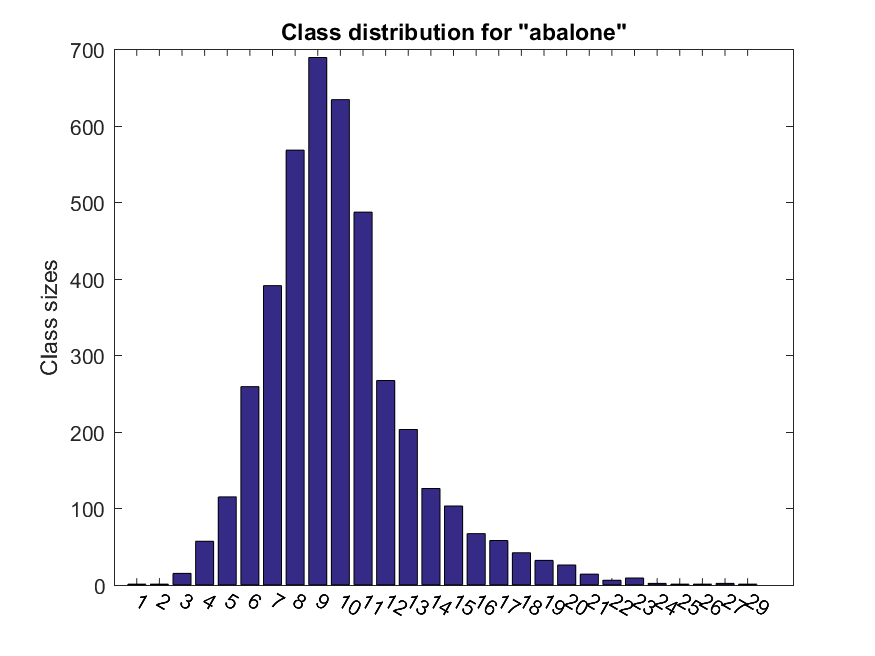We can display the feature distributions over different classes, as follows:

Example 2: abaloneClassDist01.mDS=prData('abalone'); index=DS.output>8; DS.input(:, index)=[]; DS.output(:, index)=[]; dsDistPlot(DS);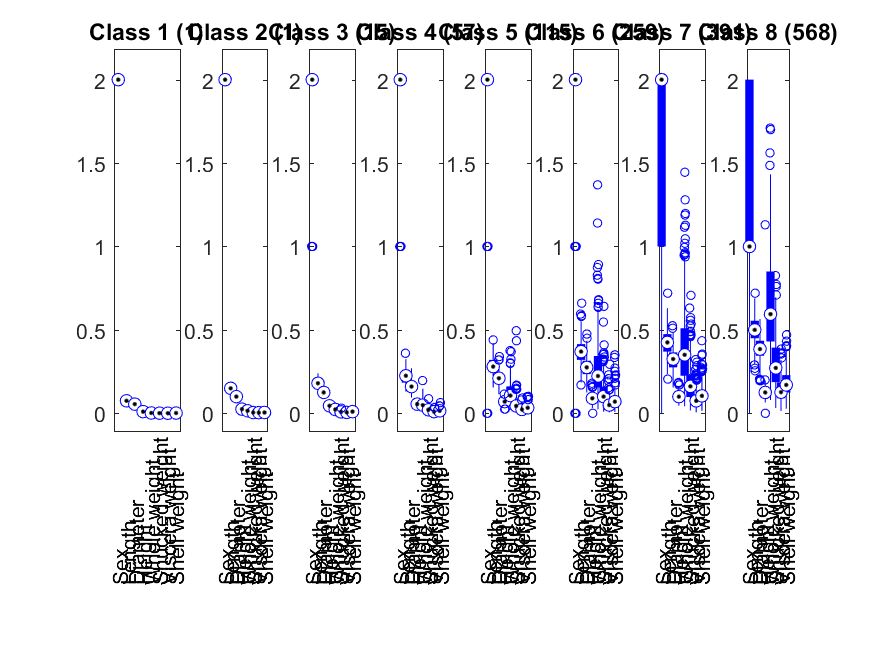(In order not to clotter the plot, we have only shown the distributions among the first 8 classes.)

We can plot the classes w.r.t. each of the features:

Example 3: abaloneProjPlot1.mDS = prData('abalone'); dsProjPlot1(DS);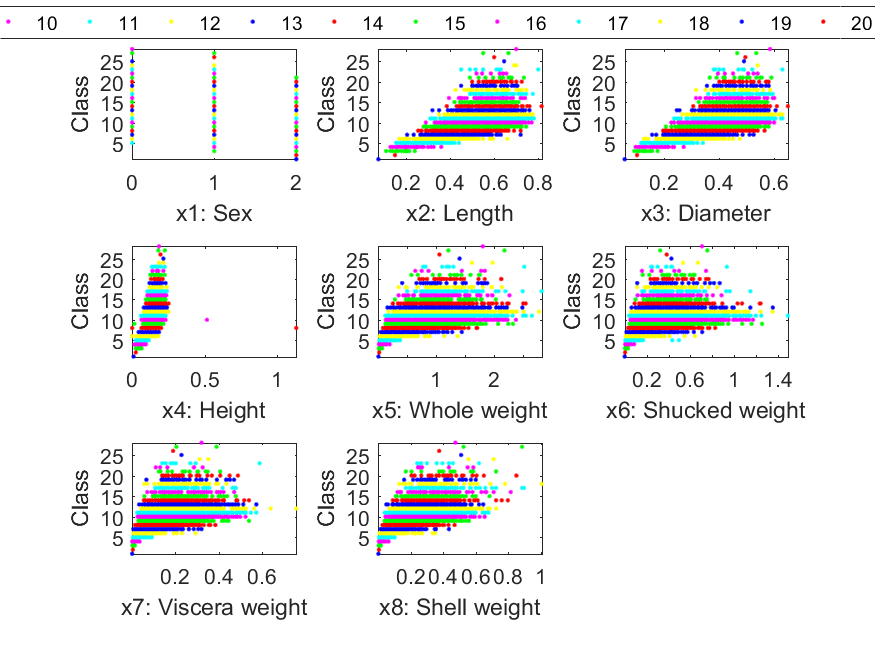We can have a scatter plot after projecting the dataset onto a 2D plane:

Example 4: abaloneProjPlot2.mDS = prData('abalone'); opt.showAxisLabel=0; opt.showAxisTick=0; opt.showClassName=1; dsProjPlot2(DS, opt);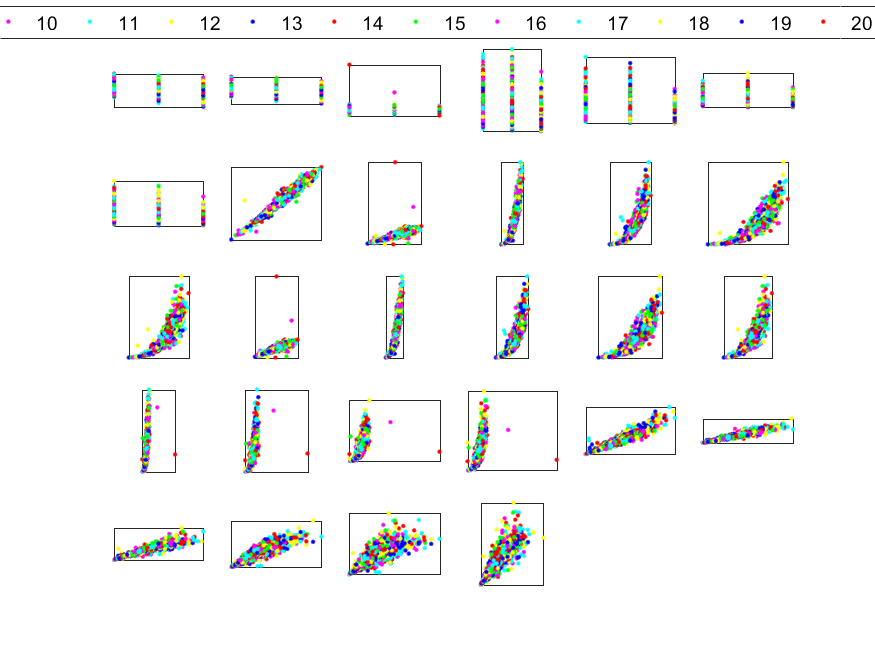We can have another scatter plot after projecting the dataset onto a 3D space, which generates C(8, 3) = 56 subplots, as follows:

Example 5: abaloneProjPlot3.mDS = prData('abalone'); opt.showAxisLabel=0; opt.showAxisTick=0; opt.showClassName=1; dsProjPlot3(DS, opt);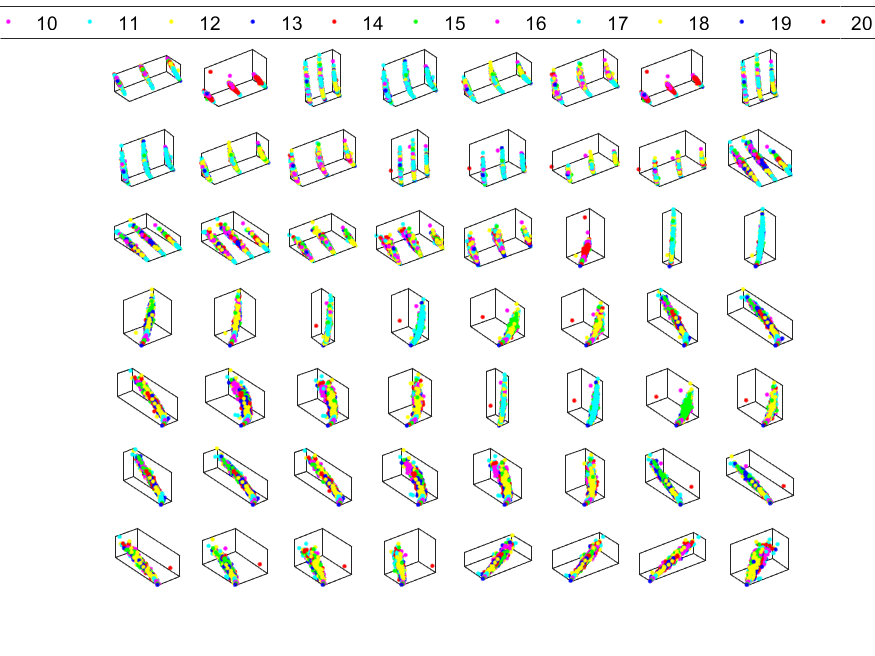Since there are 28 classes, it is hard to observe the distribution of each class in either 2D or 3D projection.

Data Clustering and Pattern Recognition (資料分群與樣式辨認)